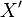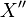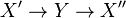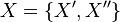# Difference between revisions of "Concept dependency tracking"

Concept dependency tracking (there might be a more standard term) refers to the tracking of conceptual dependencies (e.g. using a dependency DAG) when learning a subject.

When trying to learn a concept, there might be several conceptual dependencies (i.e. other concepts you must learn first before learning the desired concept). The nature and structure of dependencies can be simple or elaborate depending on what one is trying to learn:

• If one tries to do a "deep dive" into a subject by first picking some advanced concept (e.g. "I want to learn about Godel's incompleteness theorems") there might be multiple specific (propositional logic, first-order logic, computability) and general dependencies ("mathematical sophistication") that have some complicated structure.
• If one is following a textbook linearly or has already covered the surrounding material, then the marginal concept won't typically have elaborate dependencies. This is because the textbook is already implicitly managing the dependencies (i.e. the textbook is a serialization of some dependency graph, using for instance wikipedia:Topological sorting).

"Every time you encounter a concept you don’t recognize, you need to go back and learn it first. Pretty soon you’re deep in dependency hell, switching between twenty tabs, trying to juggle all the prerequisites of prerequisites, wondering if any of this will actually help you towards your original goal." 

The paper "Retain: Building a Concept Recommendation System that Leverages Spaced Repetition to Improve Retention in Educational Settings" by Shilpa Subrahmanyam also talks about this.

Several books have a graph near the beginning of the book describing the order in which chapters may be read.

I think most learners don't really pay attention to conceptual dependency tracking (they can just follow along in class/read the chosen-by-teachers sections in the textbook). But tracking conceptual dependencies is important for:

• Explainers who want to produce clear explanations.
• Autodidacts who get to "shop around" for multiple explanations. Different books might cover topics in a different order, so one might try reading up a topic in a different book only to find that this book assumes some other knowledge one does not have. Or the proof in the second book might circularly assume the result because it started from a different place.
• Generalists who jump from subject to subject, becoming a complete novice frequently.

## Types of dependencies

There are different "strengths" of dependencies. For example not understanding high school algebra makes it very difficult to understand calculus, so this might be a "hard" dependency. On the other hand, knowing the construction of the real number system helps to make one's understanding of real analysis solid, but is often considered nonessential during a first pass with the subject, so this might be more of a "soft" dependency.

Some subjects have feedback loops, where subject X helps shed light on subject Y, and conversely, subject Y cements one's understanding of subject X. For this kind of dependency, a DAG is insufficient. (see data structures section)

When is a dependency graph cyclic or acyclic? Circular dependencies are kind of weird in the sense that if we increase the resolution of concept space, it seems like we can always get the graph to a point where it's no longer circular. Superficially, we might say$X$ and$Y$ depend on each other, but actually, if you break them down,$X$ has parts$X'$ and$X''$, and (1)$Y$ depends on$X'$ and (2)$X''$ depends on$Y$, so at this finer resolution, the dependency has no cycle (the graph looks like$X' \rightarrow Y \rightarrow X''$), but if you look at the original graph with nodes$Y$ and$X=\{X', X''\}$, then it looks like there's a cycle. Is there a counterexample to this?

### Logical vs pedagogical dependency

In mathematics, logically speaking some axiomatic system (such as Peano axioms or ZFC) comes before everything else, and is thus a dependency. However, pedagogically speaking, it does not make any sense to start one's mathematical education with ZFC.

### Soft vs hard dependency

There are different versions/levels of the same skill, and sometimes one can get away with a fuzzy/informal/cartoonish understanding, while other times one actually needs to know something at the mechanistic/formal/instinctual level. I predict that concept dependency systems that don't take this into account will feel unpleasant to use. A related idea is Terry Tao's distinction between pre-rigorous, rigorous, and post-rigorous mathematics. Whereas Tao distinguishes between these levels for one's mathematical education as a whole, one could also take a more granular approach, where for each concept, there is a pre-rigorous, rigorous, and post-rigorous stage.

A possibly equivalent way to rephrase the above is the following: each concept has many smaller parts or affordances, and these need to be made explicit in a concept dependency tracking system. For example, "Peano axioms" isn't an atomic concept. Instead, the Peano axioms have various affordances (i.e. one can perform various actions using them; "affordance" might not be the best term), like "use the Peano axioms to prove that addition of natural numbers is commutative", "give an informal description of what the Peano axioms are", "explain how the Peano axioms are different from the axioms of Robinson arithmetic", and so on. An informal understanding of the Peano axioms would correspond to knowing fewer of these affordances, while a solid understanding corresponds to knowing more/most of these things.

## Emotional stakes

Sort of related to the logical vs pedagogical distinction, but learning requires some amount of switching back and forth between "what I care about" and "what I can easily understand given the concepts I already know". The things you emotionally care about are the big results (e.g. Godel's incompleteness theorems, singular value decomposition, how AlphaGo works), while the next thing you can easily understand are much more boring (e.g. understanding the definition of language, understanding what each axiom of Peano arithmetic does, understanding the definition of a normal operator, understanding the nth way to write the Bellman equations).

If you just crawl the dependency graph by taking the reachable nodes, it might take like 100 serial steps before you actually get to Godel's first incompleteness theorem. Maybe by that time your enthusiasm will have faded. Part of what the concept dependency system needs to do is to maintain your curiosity, by showing you interesting things (in some fuzzy/informal form) before you can fully understand them. Some sort of foreshadowing feature, or explaining the interesting idea using the abstractions that you have learned so far, every 5-20 steps of the way, or something like that. As a learner from textbooks, I already naturally do this, by flipping back and forth every chapter or so so that I can test myself: do I understand SVD yet? Am I getting any closer?

I think one of the more valuable forms of feedback an explainer can receive is the specific points of an explanation where the learner's attention starts to fade, where it gets boring.

## Examples

• Metacademy -- I don't think Metacademy distinguishes different levels of a skill/concept
• Arbital -- I think Arbital distinguishes between different levels of a skill/concept
• David R. MacIver's Current/Pending system

## Related ideas

In software engineering, the idea of dependencies is used frequently, e.g.

• Package managers for programming languages and Linux distributions
• https://en.wikipedia.org/wiki/Dependency_hell - the direct analog of this is probably less problematic in mathematics/the conceptual realm because there aren't "version numbers" on concepts and it is easy to shuffle around ideas. Terry Tao: "I think the basic reason for this is that in the purely theoretical world of mathematics, there is basically a zero cost in taking an argument that partially solves a problem, and then combining it with other ideas to make a complete solution; but in the real world, it can be difficult, costly, or socially unacceptable to reuse or recycle anything that is (or is perceived to be) even a partial failure."
• https://en.wikipedia.org/wiki/Coupling_(computer_programming)
• Tracing up and down layers of abstraction in object-oriented programming, tracing function calls -- this involves a similar mental motion of moving around in a graph

## Interactions with other ideas

A concept dependency system can interact with other kinds of "tracking" to obtain a more complete "learning scheduling system":

• Spaced repetition
• A comprehension score (see Subrahmanyam paper)
• Priority of the subject (e.g. "learning math is more important than learning physics", or "learning linear algebra is more important than learning abstract algebra")
• Current excitedness/curiosity about a subject